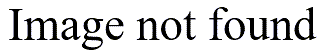# Testing multinomial proportions

## 2004-11-09

I received an email inquiry about a problem that seems simple enough, but which just doesn’t seem to have an easy answer. This person gave the following hypothetical data.

Suppose in a sample of 100 people, 21 have blue eyes and 23 have green eyes. Can you test the hypothesis that the proportion of blue eyes is equal to the proportion of green eyes?

This is not a two sample binomial problem and it is not a one sample binomial problem either. The only way you can properly analyze this data is to treat it as a single multinomial sample.

A multinomial distribution is used when your outcome variable has more than two possible values. In this data set, there are three values: blue, green, and all other colors. The problem does not change if you divide the all other colors category into finer categories (brown, hazel, pink). The formula for a multinomial probability looks just a bit messier than for a binomial probability. Here is an example when there are three categories in the multinomial distribution.The hypothesis that you want to test is that probability is the same for two of the categories in the multinomial distribution. The most obvious way to test this is to use the Chi-square goodness of fit test. The general form of this test iswhere Oi represents the observed counts and Ei represents the counts under some restrictive null hypothesis. In our situation, if the proportion of blue and green eyes are the same, then we would expect 22 in each category. So the Chi-square test computes aswhich works out to be 0.091 which you would compare to a Chi-square distribution with one degree of freedom. The p-value is 0.76, which is quite large.

You can also use a large sample normal approximation to the multinomial distribution, but you have to account for the fact that two multinomial proportions are negatively correlated. Here are the formulas for the variances and covariance.These results allow you to get an estimate of sampling error for the difference between two proportions in a multinomial distribution.Use a simple Z test to test for statistical significance.If you work this out, you get Z=0.302, which has a two-sided p-value of 0.76. It is not a coincidence that these two p-values are identical, because if you square the Z statistic, you get the Ch-square statistic.

You can also use the likelihood ratio test for this hypothesis. The NIST Engineering Statistics Handbook has a nice introduction to the likelihood ratio test. You compute the likelihood under the restricted null hypothesis (L0).Then compute the likelihood under the unrestricted general hypothesis (L1).If this ratio of L0 to L1 is close to 1, then the restrictions of the null hypothesis is reasonable. If the ratio is much smaller than 1, then the restrictions of the null hypothesis are too great to bear. In our example, the likelihood ratio reduces toIn most situations, -2 times the natural log of the likelihood ratio can be approximated as a Chi-square distribution. For our data, this produces a value of 0.091 which is again identical to the previous test and produces the same large p-value of 0.76.

Update (April 6, 2005): A sharp reader of my pages pointed out that you can recast the multinomial problem in terms of the marginal distribution, which is binomial. I had failed to recognize this and I’ll try to show how this works, with formulas, when I have time.

You can find an earlier version of this page on my original website.